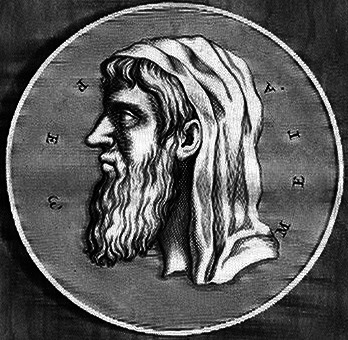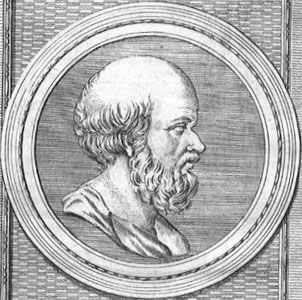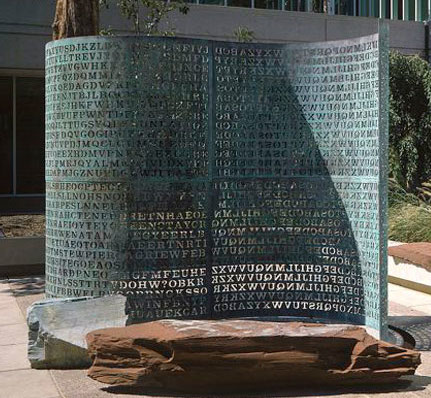Prime Numbers, i.e. numbers with no factors other than 1 and themselves, are in many ways the most important numbers of all since they cannot be simplified, cannot be broken down. They act as the building blocks for all the integers; every integer is a product of primes.

But here's the amazing part — each of these other numbers can be built from a product of primes in only one way!

Think about it. Why should this be so? Would you expect it?

For example we are perfectly happy with the fact that 84 is both 14 × 6 and 12 × 7. The numbers 6, 12 and 14 are factors of 84, but not prime factors. If we consider the prime factorisation84 = 2 × 2 × 3 × 7, the factors 2, 3 and 7 are all primes and there is no other way to get 84 from a product of primes. (Incidentally, this is why 1 is not considered prime; otherwise we could add any number of × 1 terms to a prime factorisation.)Euclid of Megara
The uniqueness of a number's prime factorisation is easy to see with smaller numbers, e.g. 15 = 3 × 5, but with a bit of work you can convince yourself it is also true of larger numbers, e.g. 747565 = 5 × 7 × 13 × 31 × 53.

Mathematicians are so amazed by this, think it is so cool, that they've given it a special name:

The Fundamental Theorem of Arithmetic

This is just a fancy way of saying "The most important property of numbers". It is a core mathematical result, proven by Euclid in 300BC.

Finding primes

Euclid also proved that there are an infinite number of primes. This proof is in his book, The Elements — the world's first maths textbook, and one that has remained in use for more than 2000 years!Eratosthenes
One way to find prime numbers is using the Sieve of Eratosthenes as follows. Starting with a list of all the integers from 2, mark 2 as prime and then remove all its multiples off the list. Then proceed to the next number in the list, in this case 3, and do the same thing. After all multiples of 2 and 3 have been removed, 4 will no longer be in the list and we must move to 5 — the next prime. Mark it as such and continue in this manner. Once you've reached the largest number you are interested in, what remains in the list are all the primes up to that number. Press the following button to give it a go:

Eratosthenes, who lived from 276 to 194BC is known as the Father of Geography. He was the first to measure the circumference of the Earth, calculating it to be 46000km (the actual value is close to 40000km). He went blind in his old age, and starved himself to death.

Many properties of primes and their relationships with other integers were discovered by the Ancient Greeks (including Euclid and Eratosthenes as we've seen). However, there are still many unsolved problems regarding primes. The most famous of these is the Goldbach Conjecture from 1742 which states that every even integer greater than 2 can be written as the sum of two primes. Extensive computer searching has failed to find a counterexample, but no one has yet managed to prove the conjecture to be true.

Cracking a code

Did you know that cracking modern day secret codes like RSA and PGP is like finding the large prime factors of an even larger number? Encoding is like multiplying two large numbers — that's easy; but decoding is like finding the factors when you only have the product. If the two large numbers are primes, that is very hard unless you have the key for the encoding — even for computers. Click the following button to have a try yourself:The Kryptos sculpture